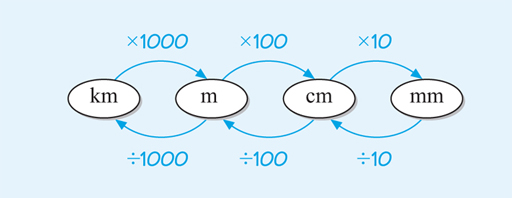Succeed with maths – Part 2

Start this free course now. Just create an account and sign in. Enrol and complete the course for a free statement of participation or digital badge if available.

Free course

# 3.1 Converting between SI units and length

You started to consider converting between different SI units of length in the last section. Take another look at Figure 1 below, showing how these relate to each other:Figure 1 Relationships between SI units of length

Notice that to convert from a physically larger unit, such as kilometre (km), to a physically smaller unit, such as metre (m), you always multiply. This makes sense – you will need a lot more of the smaller units to make one of the larger units! On the other hand, to convert a measurement in a smaller unit to a larger unit, you always divide.

Another way to think about this is whether you expect your final answer, when converting between units, to be a bigger or smaller number than the original value you had. If it should be a larger number, then multiply, if smaller, then divide.

Let’s see how this might work in an example:

A girl’s height has been measured in metres, but for a school project this needs to be shown in centimetres. Her height was measured as 1.32 metres.

Looking at Figure 1, to convert from metres to centimetres you need to multiply by 100. That makes sense, as a centimetre is a physically smaller unit than a metre, so you would expect the final answer to be a bigger number than the original value that you started with. So the

Use these ideas to have a go at this first activity of the course.

## Activity 1 SI units of length

Timing: Allow approximately 5 minutes
• a.Write 6.78 metres in centimetres.

### Comment

• a.There are 100 cm in 1 m and you are converting from a larger unit to a smaller unit, so multiply by 100.
• b.Write 327 centimetres in metres.

• b.There are 100 cm in 1 m. This time you are converting from a smaller unit to a larger unit, so divide by 100 to change cm into m. Hence,

• c.The distance to your friend’s house is about 2324 m. How far is this in kilometres?

• c.There are 1000 m in 1 km. You are converting from a physically smaller unit to a physically larger unit, so divide by 1000 to change metres into kilometres. So,

Well done, you’ve just completed your first activity for the week and the course. Many people find converting between units a tricky skill to master, but using the ideas introduced here should help you to feel more confident with this. You’ll be getting lots of practice over this week and the next to build on this as well.

The next section introduces imperial units of measurement before turning your attention back to length.